Next: 5.2.1 Surface Representation Up: 5. Surface Rate Calculation Previous: 5.1.2 Limitations

# 5.2 Ray Tracing

Another MC technique, which can be used to calculate the surface rates directly, is ray tracing [10,114]. Ray tracing is a widely applied technique in computer graphics for rendering a scene. A large number of light rays is used to obtain a realistic picture. In recent years, this MC technique has been successfully applied to topography simulation to calculate surface rates [8,64,95]. Especially if the particle trajectories are linear, which is the case for ballistic transport, the same algorithms and techniques can be applied in an analogous manner [A13,A16].

In order to simulate the particle transport and to obtain the surface rates, many particles are launched from the source plane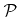, and their trajectories are calculated. Whenever a particle reaches the surface, it contributes directly to the local surface rates introduced in (2.24). For each surface point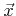, for which the rates should be calculated, a surrounding neighborhood is defined. If the area of that neighborhood, denoted by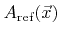, is small enough and the surface is smooth, the neighborhood can be assumed to be plane and orthogonal to the surface normal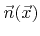. These neighborhoods of known area are necessary to relate the contribution of a single particle to the rates at point. An incident particle of type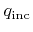with direction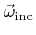and energy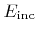striking the neighborhood of pointadds(5.13)

to the corresponding rate function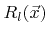. Here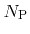denotes the total number of simulated particles. The particles are launched from the source planeand must obey the flux distribution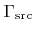(2.2).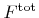is the total flux of particles through. In order to account for reemission, new particles must be launched after striking the surface. Reemitted particles need to obey the conditional probability given by the model dependent reemission probability function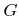. The probability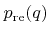that a particle of type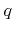is reemitted is given by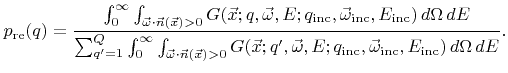(5.14)

This probability can take values larger than 1. For example, the average number of sputtered particles is often larger than 1. Hence, to satisfy statistics, multiple particles must be launched. However, this leads to a very large number of secondary particles which all must be simulated.

A better strategy is to introduce a volume or weight factor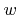assigned to a particle as proposed in  and which allows to control the number of reemitted particles. Initially, when a particle is launched from the source, its weight factor is set to unity (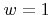). An incident particle contributes to the local rates according to its current weight factor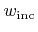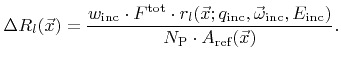(5.15)

Instead of reemitting several particles of typeaccording to the probability, it is possible to initialize just one new particle with an adequate weight factor calculated as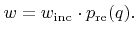(5.16)

The weight factor can be used as termination criterion. If the weight factor is below a certain critical level, so that the contribution to the surface rates is negligible, the reemission of new particles can be avoided [A20].

The directional and energy distribution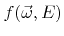of a reemitted particle speciesobeys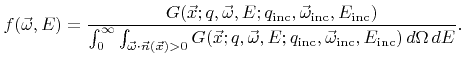(5.17)

The accuracy of the statistically calculated rates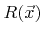is primarily influenced by the average number of incident particles on a neighborhood, which is proportional to the total number of simulated particlesand the corresponding area. To improve the accuracy, either more particles must be simulated or the areas of the neighborhoods must be increased at the expense of the spatial resolution.

The main computational task of this ray tracing technique is to determine the first surface intersection of rays and to test for intersections with the neighborhoods of all surface points, for which the surface rates should be calculated. The choice of surface representation and of neighborhoods, as well as the corresponding intersection tests are discussed in the following sections.

SubsectionsNext: 5.2.1 Surface Representation Up: 5. Surface Rate Calculation Previous: 5.1.2 Limitations

Otmar Ertl: Numerical Methods for Topography Simulation